Previous: Scaling Objects  Up: Selecting and Editing Objects  Next: Distorting Shapes

# Shearing Objects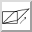Selected objects can be sheared either by clicking on the shear button or by selecting Transform->Shear (Ctrl-H). This will open up a dialog box in which you can specify the shear factors. The shearing transformation is given by:

 ⎛ 1 𝑠𝑥 ⎞ ⎛ 𝑥 ⎞ ⎛ 𝑥+𝑠𝑥𝑦 ⎞ ⎜ ⎟ ⎜ ⎟ = ⎜ ⎟ ⎝ 𝑠𝑦 1 ⎠ ⎝ 𝑦 ⎠ ⎝ 𝑦+𝑠𝑦𝑥 ⎠

Notes:

• Individual objects will be sheared relative to the bottom left corner of the object's bounding box.

• Objects within a group will be sheared relative to the bottom left corner of the group's bounding box.

• Shearing a text-path will shear the path and the text will adjust to follow the transformed path. Note that the text itself will not be sheared.

• Shearing a text area and path and then combining them to form a text-path is not the same as first combining and then shearing.

• Shearing a pattern will shear the underlying path not the complete shape.

To illustrate this, in Figure 8.23(a) there are three objects selected. The selection is then sheared with shear factors sx=1 and sy=0. The result is shown in Figure 8.23(b).

In Figure 8.24, the three objects in Figure 8.23 were first grouped (Figure 8.24(a)) and then sheared with shear factors sx=1 and sy=0 (Figure 8.24(b)).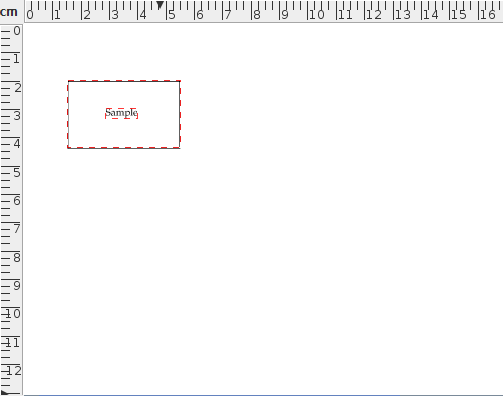(a)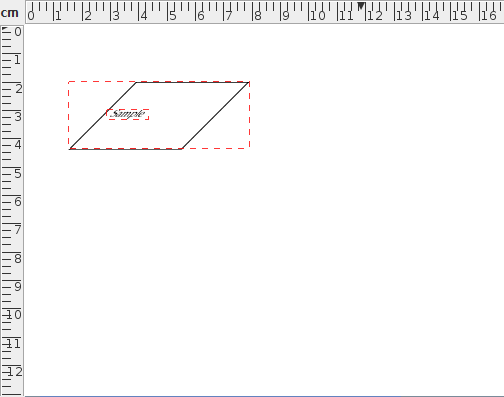(b)
Figure 8.23: Two selected objects sheared horizontally: (a) before, (b) after.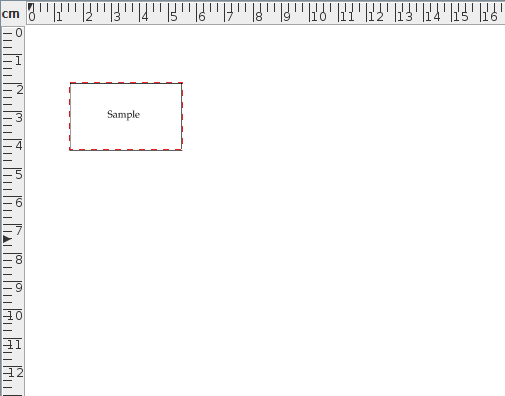(a)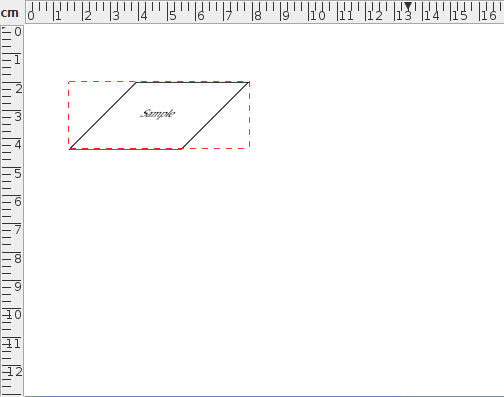(b)
Figure 8.24: A group consisting of two objects sheared horizontally: (a) before, (b) after.

In Figure 8.25, the path and text area in Figure 8.25(a) are combined into a text-path, shown in Figure 8.25(b). This text-path is then sheared with shear factors sx=1 and sy=0 Figure 8.25(c). Note that this is different from first shearing the original path and text area, shown in Figure 8.25(d), and then combining them to form a text-path, shown in Figure 8.25(e).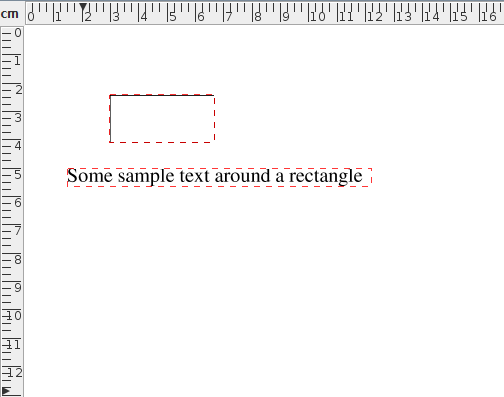(a)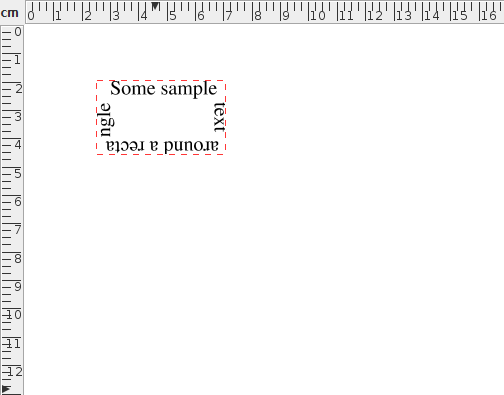(b)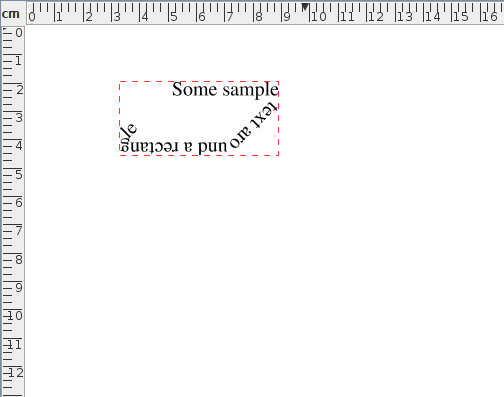(c)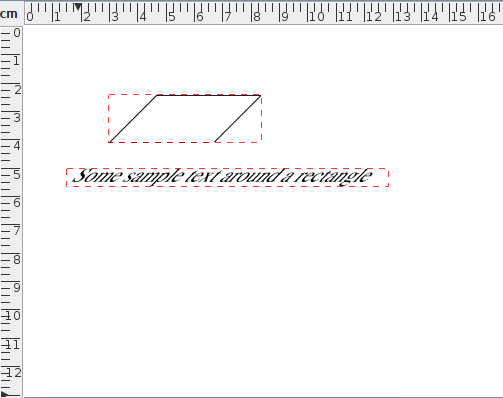(d)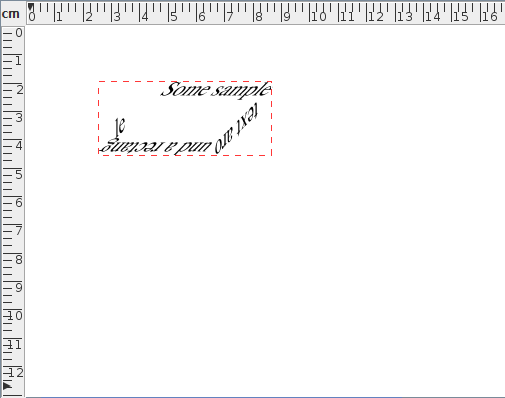(e)
Figure 8.25: Shearing a text-path: (a) original text area and path; (b) text area and path in (a) combined to form a text-path; (c) text-path in (b) sheared horizontally; (d) text area and path in (a) sheared horizontally; (e) sheared text area and path in (d) combined to form a text-path.

In Figure 8.26(a), a path created using the ellipse tool has been converted into a rotational pattern with two replicas. This pattern is then sheared with shear factors sx=1 and sy=0, shown in Figure 8.26(b). This is different from the effect obtained by applying the same shear factors to a complete path rather than a pattern. Figure 8.26(c) is a full path version of Figure 8.26(d). This path is then sheared using the same factors and the result is shown in Figure 8.26(d).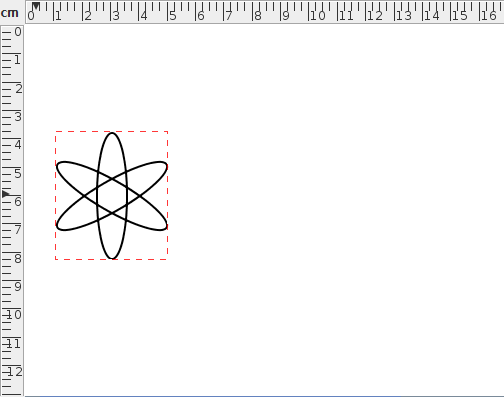(a)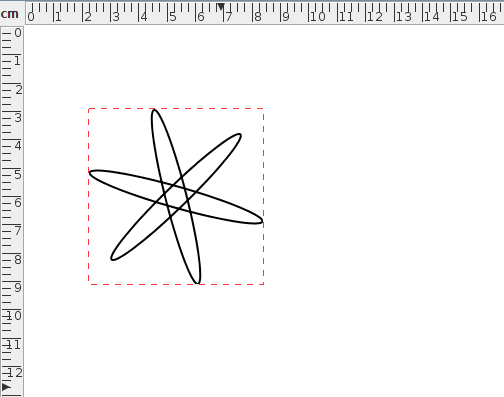(b)(c)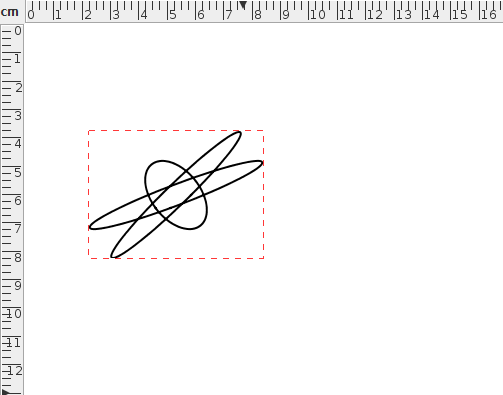(d)
Figure 8.26: Shearing a pattern: (a) a pattern consisting of an ellipse that has 2 rotational replicas; (b) the pattern in (a) has been sheared horizontally; (c) the pattern in (a) has been converted to a full path; (d) the path in (c) has been sheared horizontally.

If you prefer to shear an object using the mouse, you first need to enable the hotspots. Then drag the top right hotspot to shear vertically or the top left hotspot to shear horizontally. Note that even if you have more than one object selected, only the object whose hotspot you are dragging will be transformed.